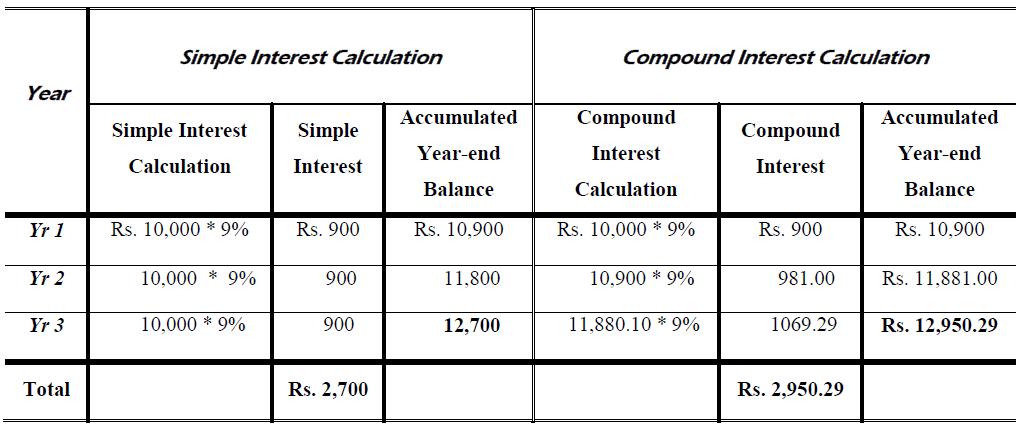Select Page

Future Value of a Single Amount Problems and Solutions

Problem 1: Simple interest and compound interest

Calculate simple interest and compound interest assuming that principal amount is Rs. 10,000; interest rate is 9% for three years. What is the amount different between compound and simple interest?

Solution:Difference = 2,950.29 – 2,700 = Rs. 250.29

>> Other Related Practice Finance Problems

Problem 2: Future value of money

You are scheduled to receive Rs. 13,000 in two years.  When you receive it, you will invest it for six more years at 8 percent per year.  How much will you have in eight years?

Solution:

13,000 (1 + 0.08) 6

>> More Practice Present Value Problems

Problem 3: Future value intra-year compounding

You have Rs. 9,000 to deposit.  ABC Bank offers 12 percent per year compounded monthly, while King Bank offers 12 percent but will only compound annually.  How much will your investment be worth in 10 years at each bank?

Solution:

ABC Bank

9,000 (1 + 0.12/12) 10 * 12

King Bank

9,000 (1 + 0.12) 10

Variance Rs. 1,750.85

Problem 4: Future value of a single amount

You invest Rs. 10,000.  During the first year the investment earned 20% for the year.  During the second year, you earned only 4% for that year.   How much is your original deposit worth at the end of the two years?

Solution:

FV =10,000 (1.20) (1.04)

Problem 5: Simple interest

Ali deposited Rs. 2,500 in an account that pays 6% simple interest.  How much money will he have at the end of 3 years?

Solution:

2,500 + (2,500 * 0.06 * 3)

Problem 6: Future value annual compounding

What is the future value of Rs. 26 invested for 32 years at an average rate of return of 7%?

Solution:

FV           =    26 (1.07) 32

Problem 7: Different cases of compounding

If interest is compounded quarterly, how much will you have in a bank account?

(a)  If you deposit today Rs. 8,000 at the end of 3 months, if the bank pays 5.0% APR?

Solution:

8,000 (1 + 0.05 / 4)

(b)  If you deposit today Rs. 10,000 at the end of 6 months, if the bank pays 9.0% APR?

Solution:

10,000 (1 + 0.09 / 4) 2

(c) If you deposit today Rs. 80,000 at the end of 12 months, if the bank pays 8.0% APR?

Solution:

80,000 (1 + 0.08 / 4) 1 * 4

(d) If you deposit today Rs. 5,000 at the end of 24 months, if the bank pays 5.0% APR and compound monthly?

Solution:

5,000 (1 + 0.05 / 4) 2 * 4

Problem 8: Future value based on flexiable interest rates

Find the future value of Rs. 100,000 for 15 years. The current five-year rate is 6%.  Rates for the second and third five-year periods and expected to be 6.5% and 7.5%, respectively.

Solution:

FV           =              100,000 (1.06)5(1.065)5(1.075)5

FV           =              100,000 (1.3382) (1.37009) (1.43563)

FV           =              100,000 (2.6322)

Problem 9: Compounding based on flexiable interest rates

The Green Corporation needs Rs. 50 million to repay a loan which is due at the end of seven years. If Green makes the following sinking fund payments, will there be sufficient funds available to repay the loan on schedule?

Solution:

FV of the Rs. 12 million = 12(1.11)7 = Rs. 24.9139

FV of the Rs. 10 million = 10 (1.1)5 = Rs. 16.1051

FV of the Rs. 8 million = 8(1.09)3 = Rs. 10.3602

Answer:  Rs. 51.382 Million

Problem 10: Future value of single amount

If farm land is currently worth Rs. 1,750 per acre and is expected to increase in value at a rate of 5 percent annually, what will it be worth in 5 years?  In 10 years?  In 20 years by factor formula and table?

Solution:

5 years:  Rs. 1,750 * 1.2763